Plotting Data

# Plotting Data

In this lesson, we will learn how to make simple plots of numeric and factorial data. We will begin to modify the defaults of these plots. As with most stats software the default options are not great. Usually, you will want to modify your plots, either to fit the publication requirements of a journal, or to make the plots objectively ‘better’, in line with recommended best practice.

We will be using the base plotting system and talk more about how you can exploit all its many parameters to get the plot you want.

Moreover, we’ll focus on using the base plotting system to create graphics on the screen device rather than another graphics device.

The base system is very intuitive and easy to use. It is akin to drawing a plot using a pencil and ruler, building up the elements piece by piece.

You can’t go backwards, though, say, if you need to readjust margins or have misspelled a caption. To go ‘backwards’, you would need to start drawing the whole plot again from scratch (i.e., re-running the code chunk—not normally a big deal). A finished plot will therefore be a series of R commands, so it will be difficult to translate a finished plot into a different system." Calling a basic routine such as plot(x, y) or hist(x) launches a graphics device (if one is not already open) and draws a new plot on the device. A graphics ‘device’ is just a general word for either the screen, or a graphics file such as .pdf or .png.

Let’s try our hand at some simple graphs using a small dataset on sparrow morphology. It is loaded with this lesson. Have a look at it. Type ‘BirdData’.

``````BirdData <- data.frame(
Tarsus  = c(22.3, 19.7, 20.8, 20.3, 20.8, 21.5, 20.6, 21.5),
Head    = c(31.2, 30.4, 30.6, 30.3, 30.3, 30.8, 32.5, 31.6),
Weight  = c(9.5, 13.8, 14.8, 15.2, 15.5, 15.6, 15.6, 15.7),
Wingcrd = c(59, 55, 53.5, 55, 52.5, 57.5, 53, 55),
Species = c('A', 'A', 'A', 'A', 'A',  'B', 'B', 'B')
)
BirdData``````
``````##   Tarsus Head Weight Wingcrd Species
## 1   22.3 31.2    9.5    59.0       A
## 2   19.7 30.4   13.8    55.0       A
## 3   20.8 30.6   14.8    53.5       A
## 4   20.3 30.3   15.2    55.0       A
## 5   20.8 30.3   15.5    52.5       A
## 6   21.5 30.8   15.6    57.5       B
## 7   20.6 32.5   15.6    53.0       B
## 8   21.5 31.6   15.7    55.0       B``````

We will start by using the generic `plot()` function, which does various things depending what data you input. The function `plot()` is a wrapper for specific `plot()` functions that take over if criteria (such as the class of the input data) are met.

You can plot a single vector/column, and it will appear in the same order as the data. Use `plot()` to plot the `\$Head` column.

``plot(BirdData\$Head)``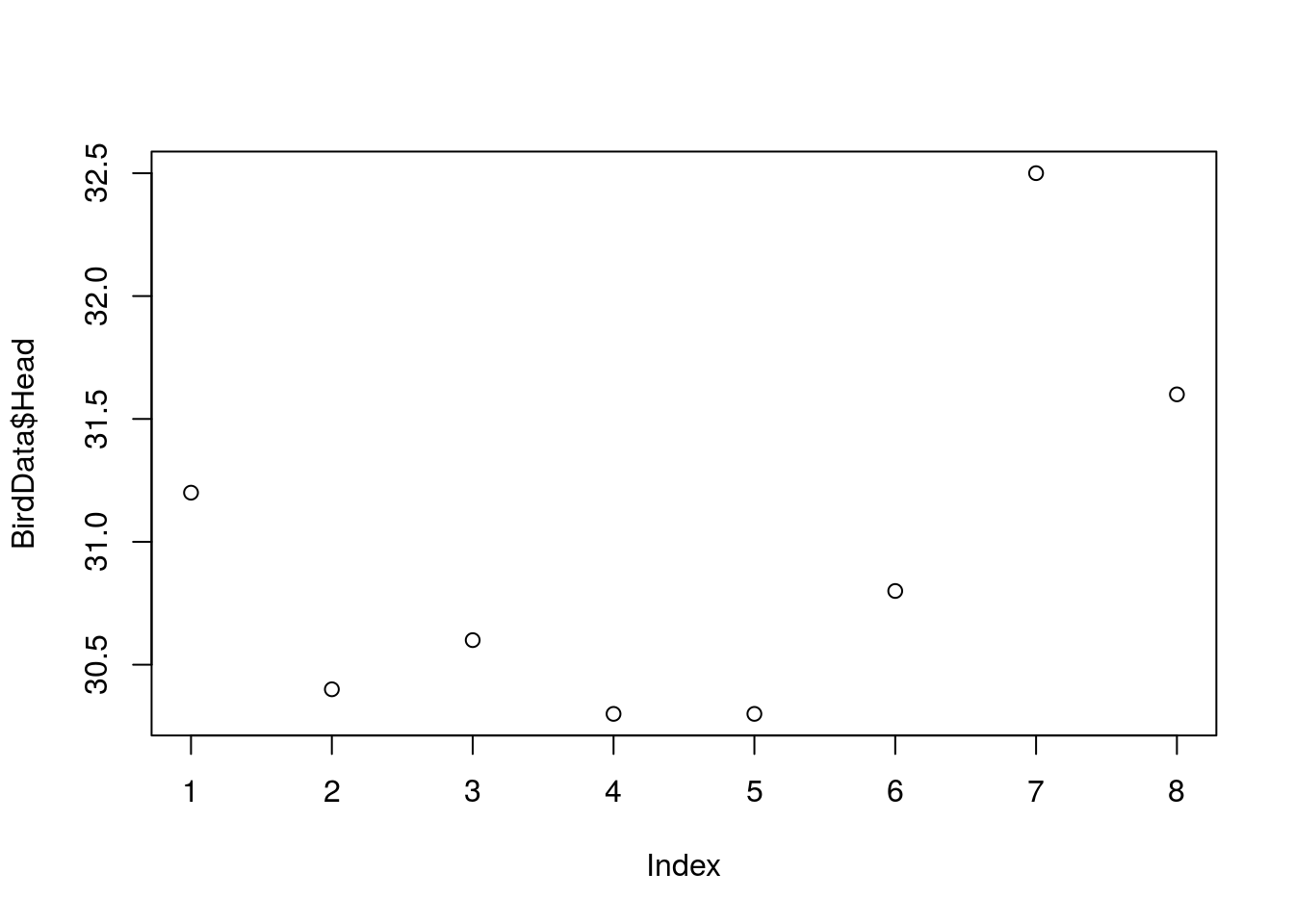You can see that the value of each circle is given along the y-axis. The axis has the label ‘BirdData\$Head’. The points are also in order they appear in the dataset, i.e., according to their ‘index’.

This figure illustrates many of the defaults in the base plotting system in R. Axes have labels based on the data you feed in; everything is in the same font; labels and numbers run parallel to their axis; tick marks lie outside the lines; data points are empty circles.

We will come back to these defaults shortly. For now, let’s see what happens when we input different data.

How about if we wanted to arrange our data in order of their `\$Head` size, rather than the order they appear in the data? Use the function `sort()` to display these data.

``sort(BirdData\$Head)``
``##  30.3 30.3 30.4 30.6 30.8 31.2 31.6 32.5``

Now, plot a figure as before, but with the sorted data nested within the call to `plot()`.

``plot(sort(BirdData\$Head))``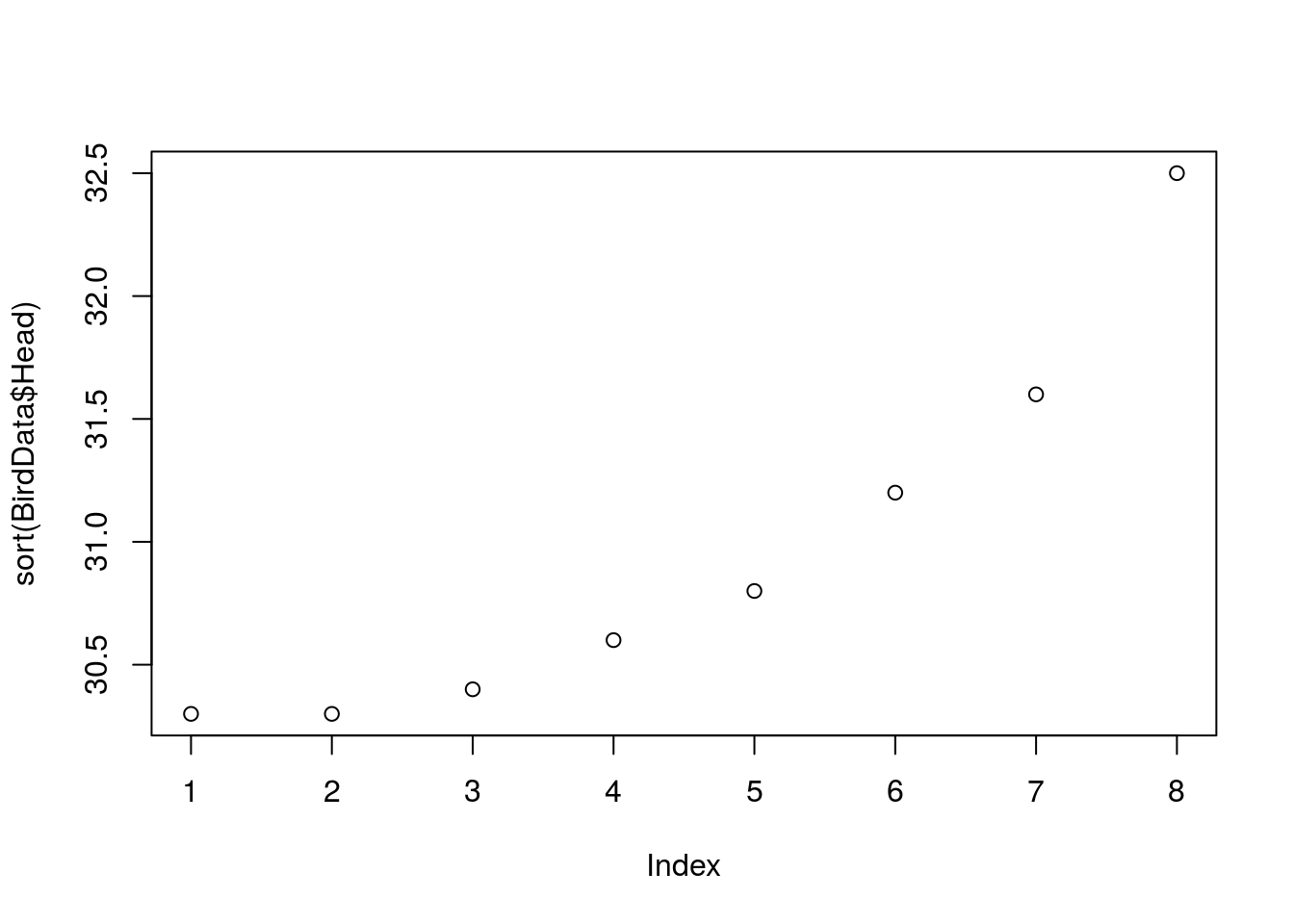Plotting a single numeric variable plots each data point. What does plotting a single categorical variable with `plot()` do? Try it out… use `plot()` on the `\$Species` column.

``plot(BirdData\$Species)``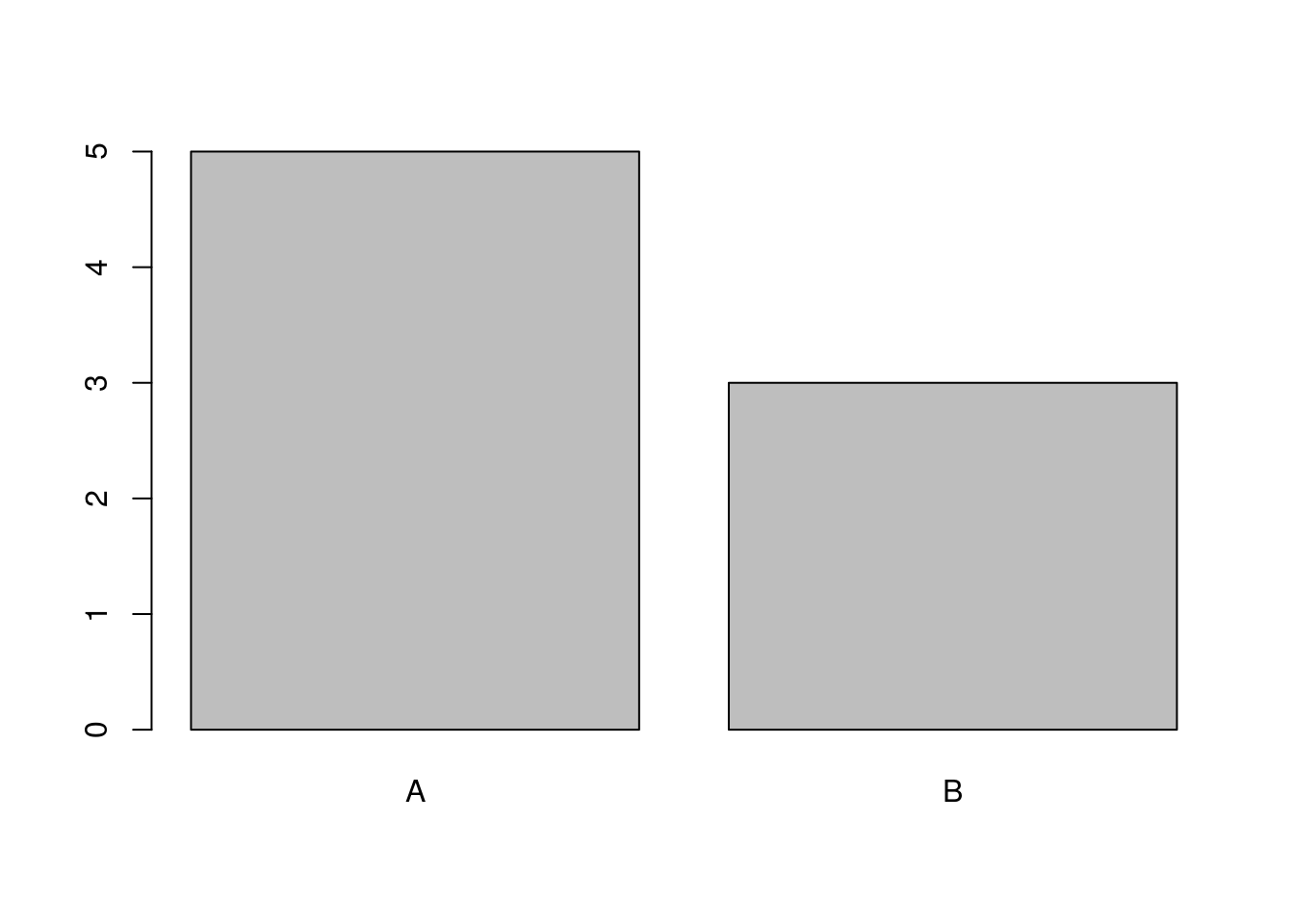Plotting a factor results in counts of each level, much like the function `table()`. It is a kind of barplot.

Lovely. You can plot one variable! However, most graphics are used to look at the relationships between two or more variables.

Let’s try plotting two numeric columns first. Plot `\$Head` as a function of `\$Tarsus`. Use a formula to do so: `BirdData\$Head ~ BirdData\$Tarsus`.

``plot(BirdData\$Head ~ BirdData\$Tarsus)``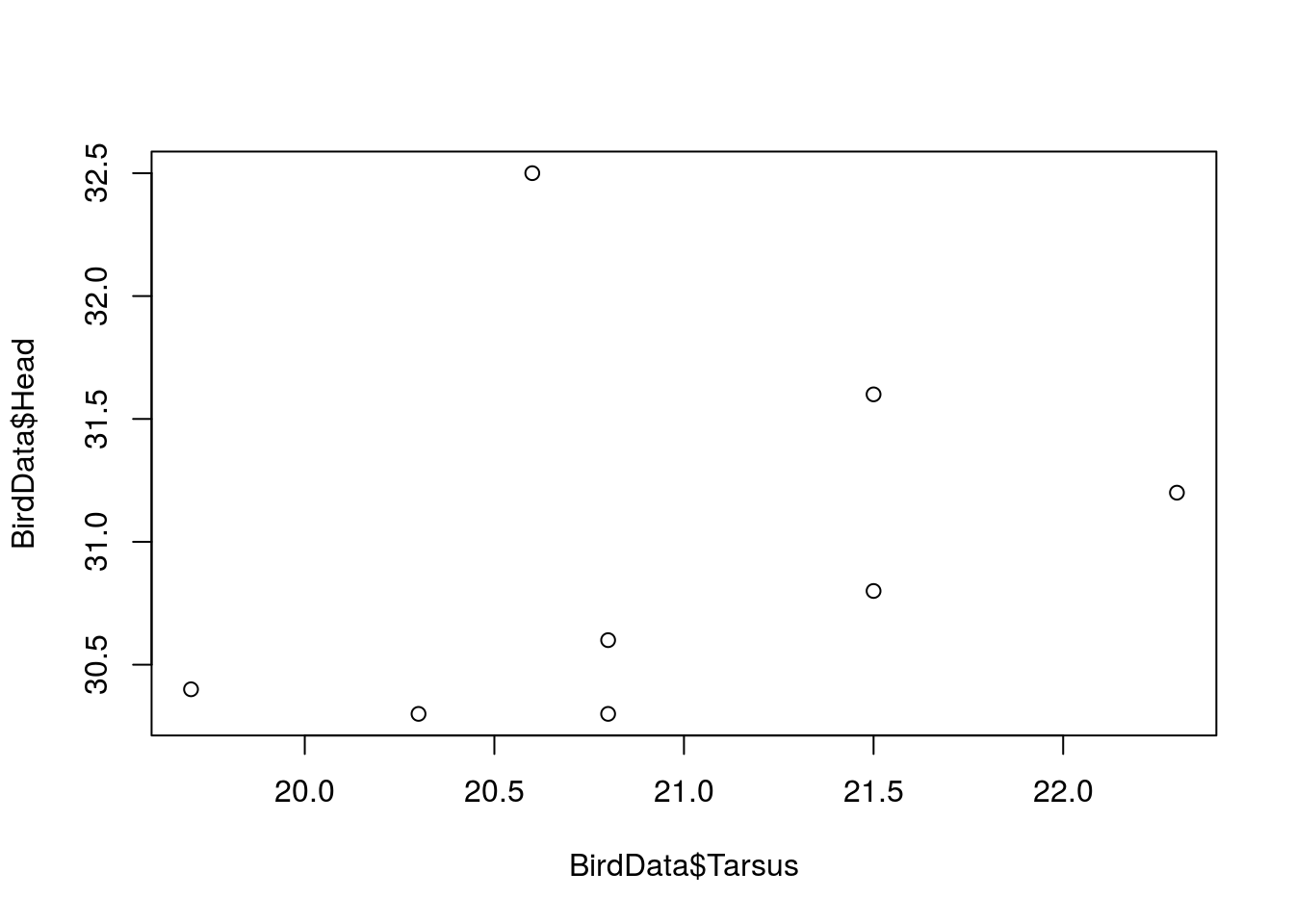Plotting two numeric columns makes a scatterplot. What about a numeric variable as a function of a factor? Plot `\$Head` as a function of `\$Species`.

``plot(BirdData\$Head ~ BirdData\$Species)``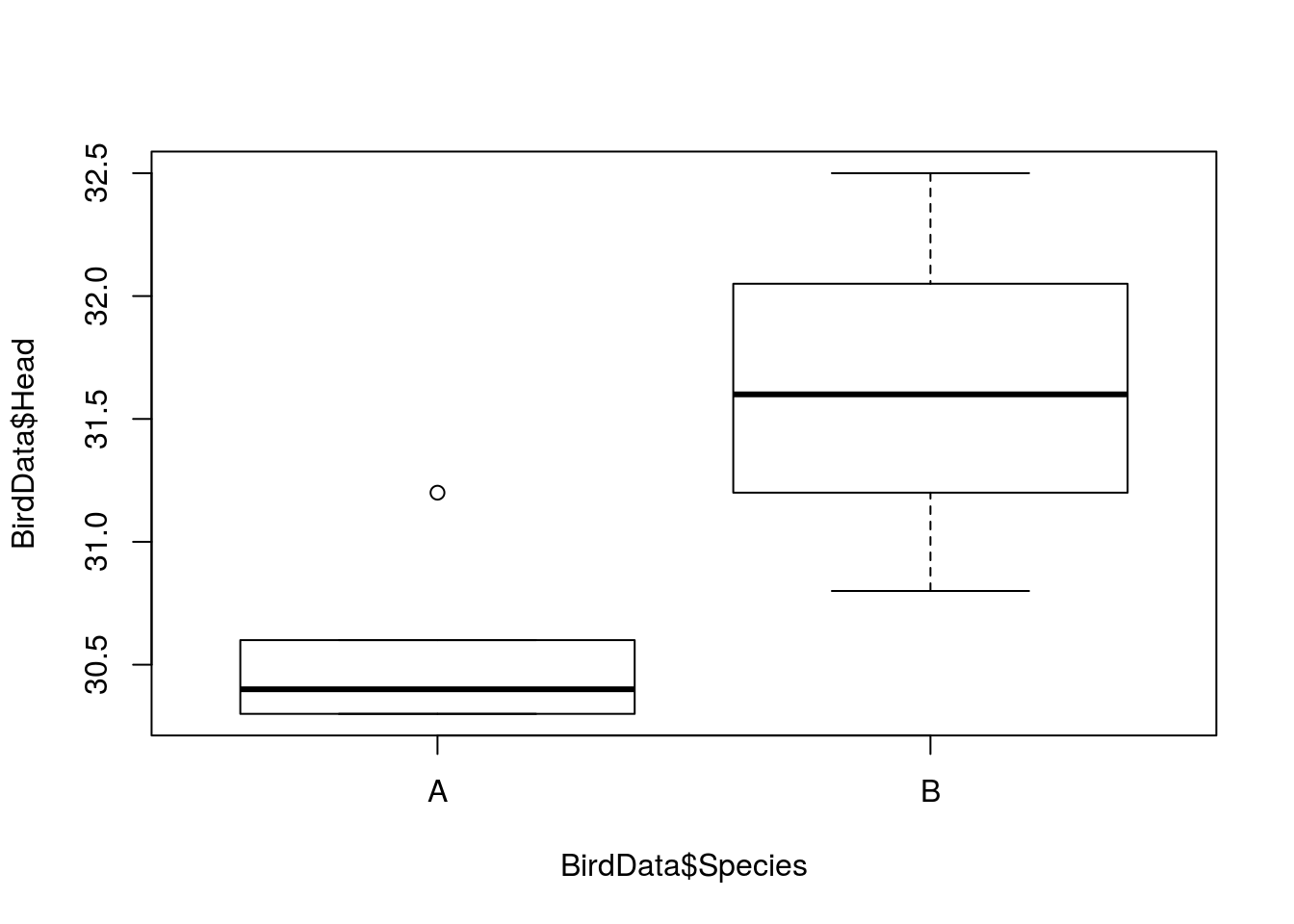A numeric and a factor makes a boxplot.

Now that you can use `plot()` to make different graphs depending on the input, let’s explore the arguments to `plot()` in more detail.

We have already used a formula structure to tell `plot()` what data we want to plot. The tilde (`~`) in R formula notation means ‘as a function of’. The y variable goes to the left side, and the x variable on the right.

If you look at the help page for `plot()`, you will see that the only two required arguments to use in `plot()` are `x` and `y`: the data arguments, i.e., the x- and y-axes. In fact, the y argument is optional. In the case of the formula, that takes the x argumment and so we do not need y.

However, in lieu of writing a formula these two arguments can be specified explicitly. Set ‘x’ to BirdData\(Species and 'y' to BirdData\)Head.

``plot(x = BirdData\$Species, y = BirdData\$Head)``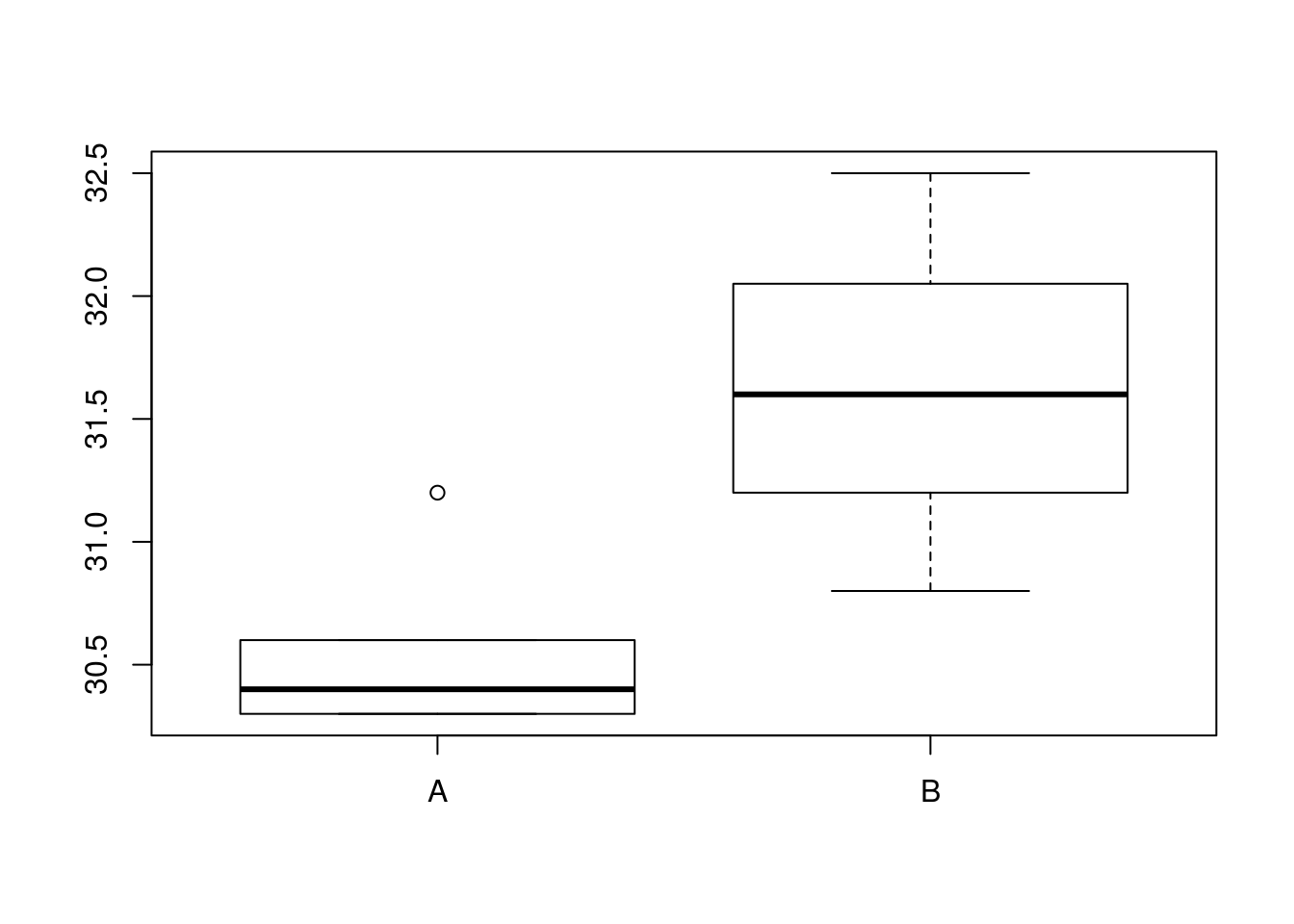Note that in this case, R does not include axis labels.

In many cases, using the formula approach will be slightly easier to understand at a glance, because it is more obvious which variable is being plotted as a function of the other.

We can make things even easier to read and understand if we use the `data =` argument. Use the arrow keys to scroll up to the previous command that created the boxplot, using a formula. Delete ‘BirdData\$’ from before both ‘Head’ and ‘Species’. Add the name of the data object to the argument `data =`, nested within the `plot()` function.

``plot(Head ~ Species, data = BirdData)``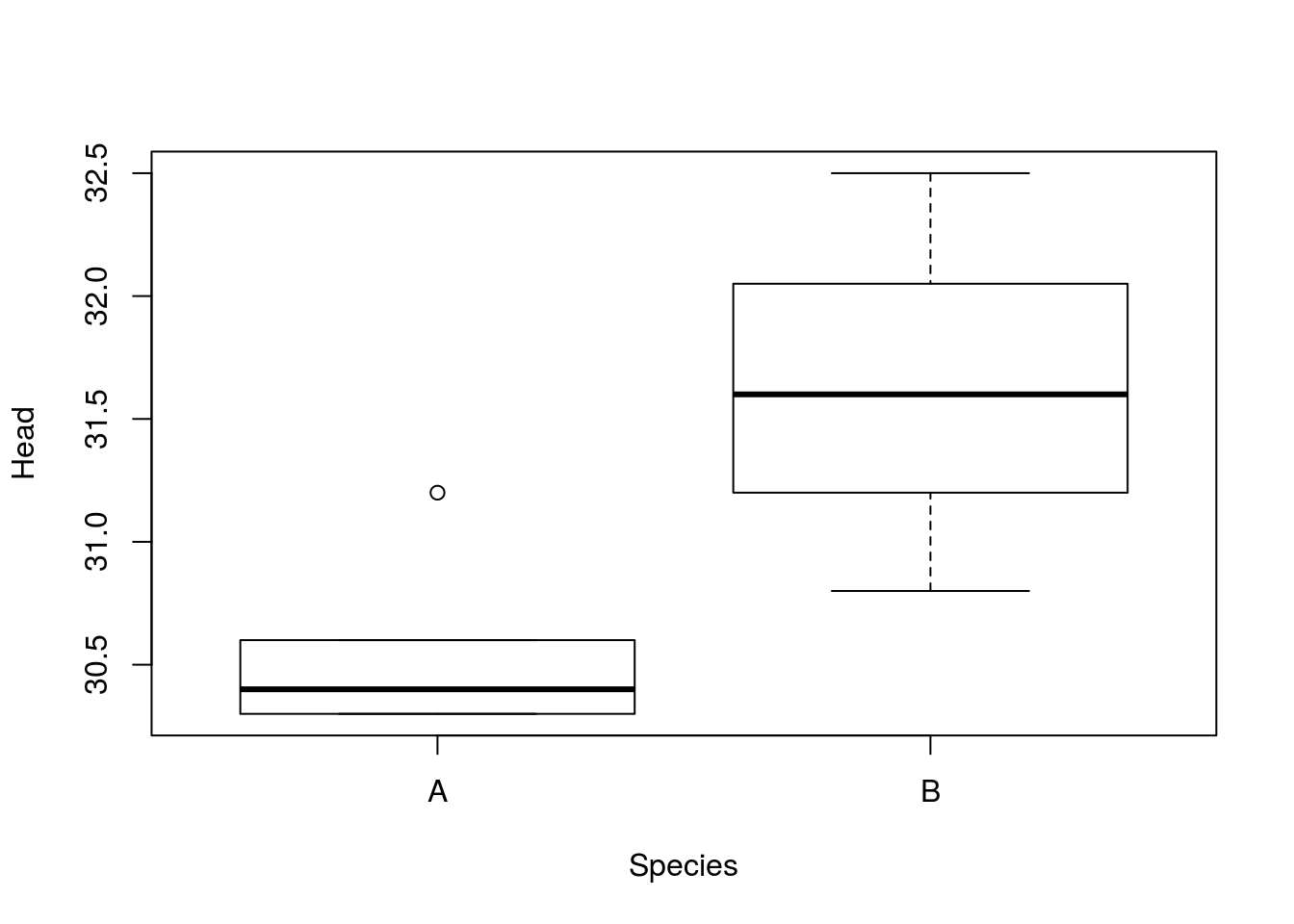Wow! This code is almost understandable as a regular language! Ok, so here it should be clear that we are plotting Head size as a function of Species, both of which come from the BirdData data object.

This `data =` argument is only available if you use the formula approach to input data to `plot()`. The `plot()` function calls the `plot.formula()` function. Check the help pages if you want.

Another advantage of the `data =` argument, apart from making the code a bit clearer, is that it then is easier to manipulate the data object from within the call to `plot()`.

For example, if we wanted to subset out the first four sparrows, we could either first create a new data object which is passed to plot: `BirdData1 <- BirdData[1:4, ]`.

Or, we could subset both `\$Head` and `\$Species` individually: `plot(BirdData\$Head[1:4] ~ BirdData\$Species[1:4])`.

Both these approaches create extra steps or require repetition, where errors could creep in. It would be much better to use a single location where we subset that applies to both variables that we want to plot.

Plot `\$Head` as a function of `\$Tarsus`, using the `data =` argument of `plot()` to subset out the first four sparrows.

``plot(Head ~ Tarsus, dat = BirdData[1:4, ])``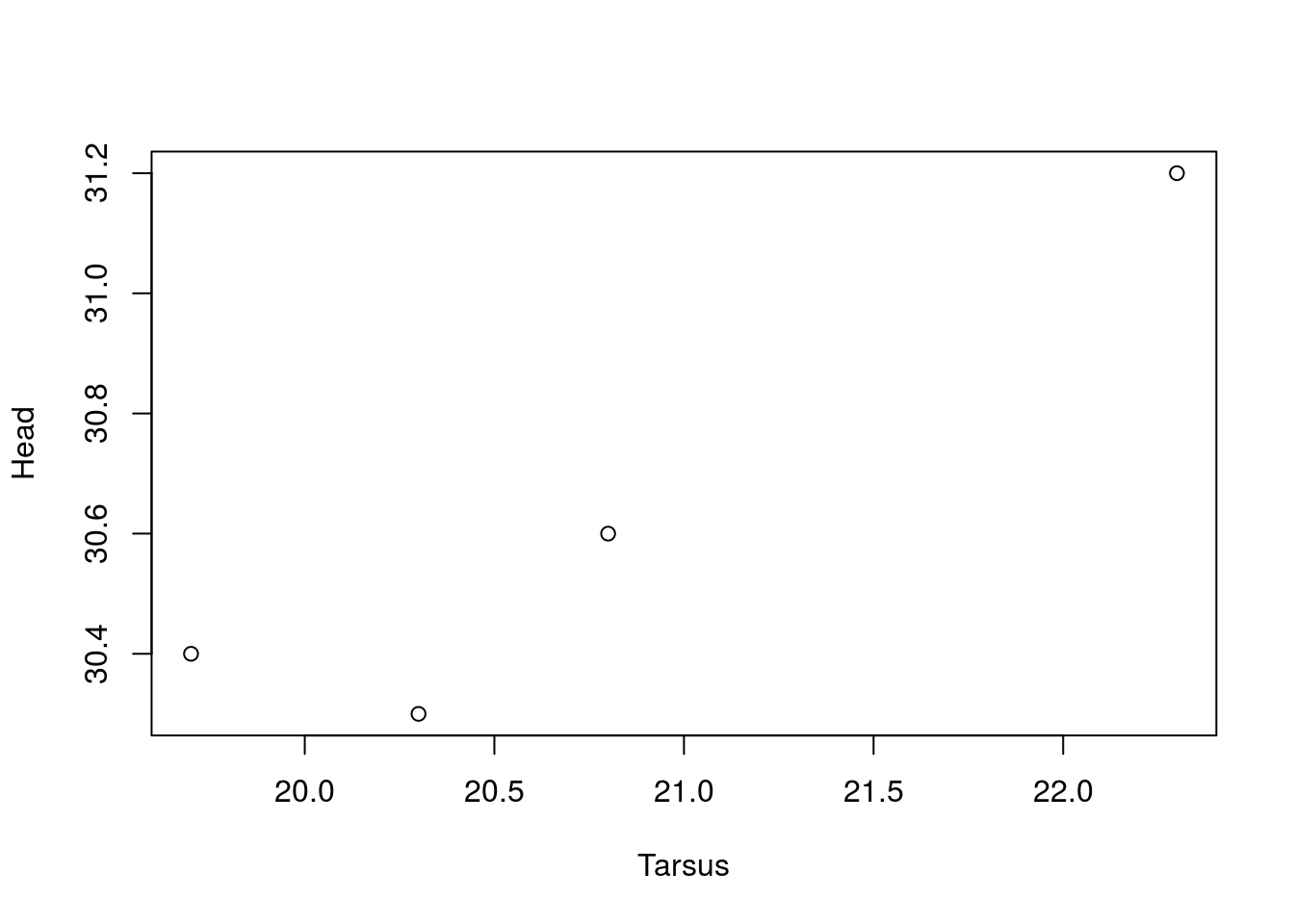Alternatively, we could sort the whole dataframe by one of the columns, to plot the points in a different order. We used the function `sort()` to change the arrangement of a single variable when we began this lesson. To sort an entire dataframe is a bit more complex.

Instead of `sort()`, we would use the function `order()`. This function returns the indices of a variable, in ascending order of their value. Look at what happens when you run `order()` on BirdData\$Tarsus.

``order(BirdData\$Tarsus)``
``##  2 4 7 3 5 6 8 1``

Now, try using this order expression inside `[ ]` square brackets to subset the original data.

``BirdData[order(BirdData\$Tarsus), ]``
``````##   Tarsus Head Weight Wingcrd Species
## 2   19.7 30.4   13.8    55.0       A
## 4   20.3 30.3   15.2    55.0       A
## 7   20.6 32.5   15.6    53.0       B
## 3   20.8 30.6   14.8    53.5       A
## 5   20.8 30.3   15.5    52.5       A
## 6   21.5 30.8   15.6    57.5       B
## 8   21.5 31.6   15.7    55.0       B
## 1   22.3 31.2    9.5    59.0       A``````

Placing this ordered vector inside the brackets is a essentially subsetting all the data. It is akin to one of the uses of `sample()`, where we sampled the entire vector without replacement, essentially shuffling everything into a random order. However, with `order()`, we have a spceific order in mind. The indices of the vector (the column `\$Tarsus`) then are used as the row numbers across the entire dataset.

You can see that the whole data object ‘BirdData’ is now sorted by the Tarsus column, which goes from 19.7 to 22.3, compared to the unsorted BirdData.

Now plot this ordered dataset in a call to `plot()`, for Head size as a function of Tarsus.

``plot(Head ~ Tarsus, data = BirdData[order(BirdData\$Tarsus), ])``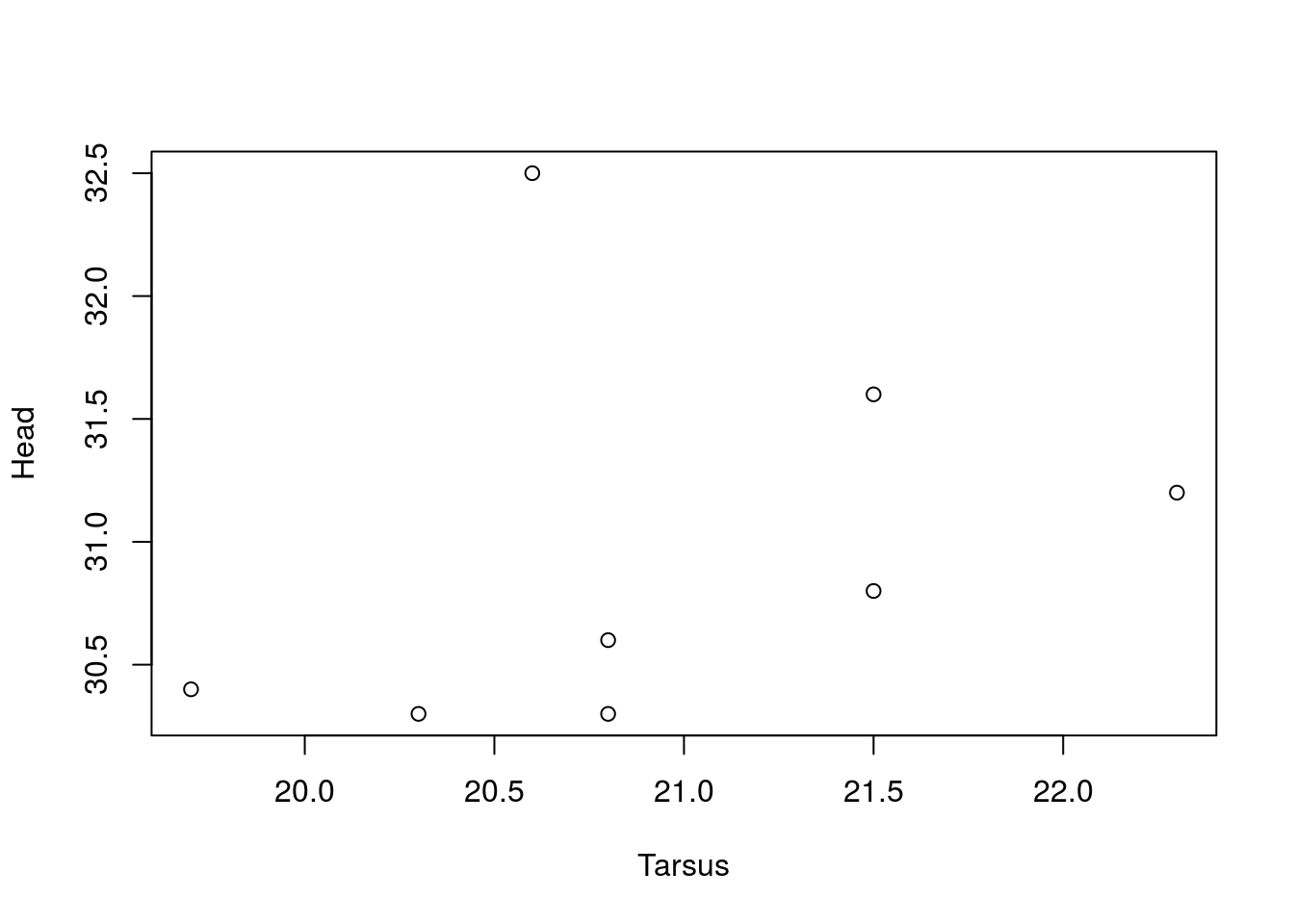Now that you can create different types of plots with different parts of a data object, let’s look at modifying the default plot.

The default plot in R is not too bad (better than Excel, at least!), but does require some modification for publication. Given that the default plot() only has two arguments, all subsequent arguments must be specified explicitly.

With two numeric variables the default plot is a scatterplot. What if we wanted a line plot instead (i.e., a joined up line that goes from each x-y point)? The argument `type =` can be used to set the kind of plot, including `type = 'l'` (a line plot), `type = 'b'` (both points and lines), as well as `type = 'n'` (for no plotting).

Make a line plot of Head on Tarsus.

``plot(Head ~ Tarsus, data = BirdData, type = 'l')``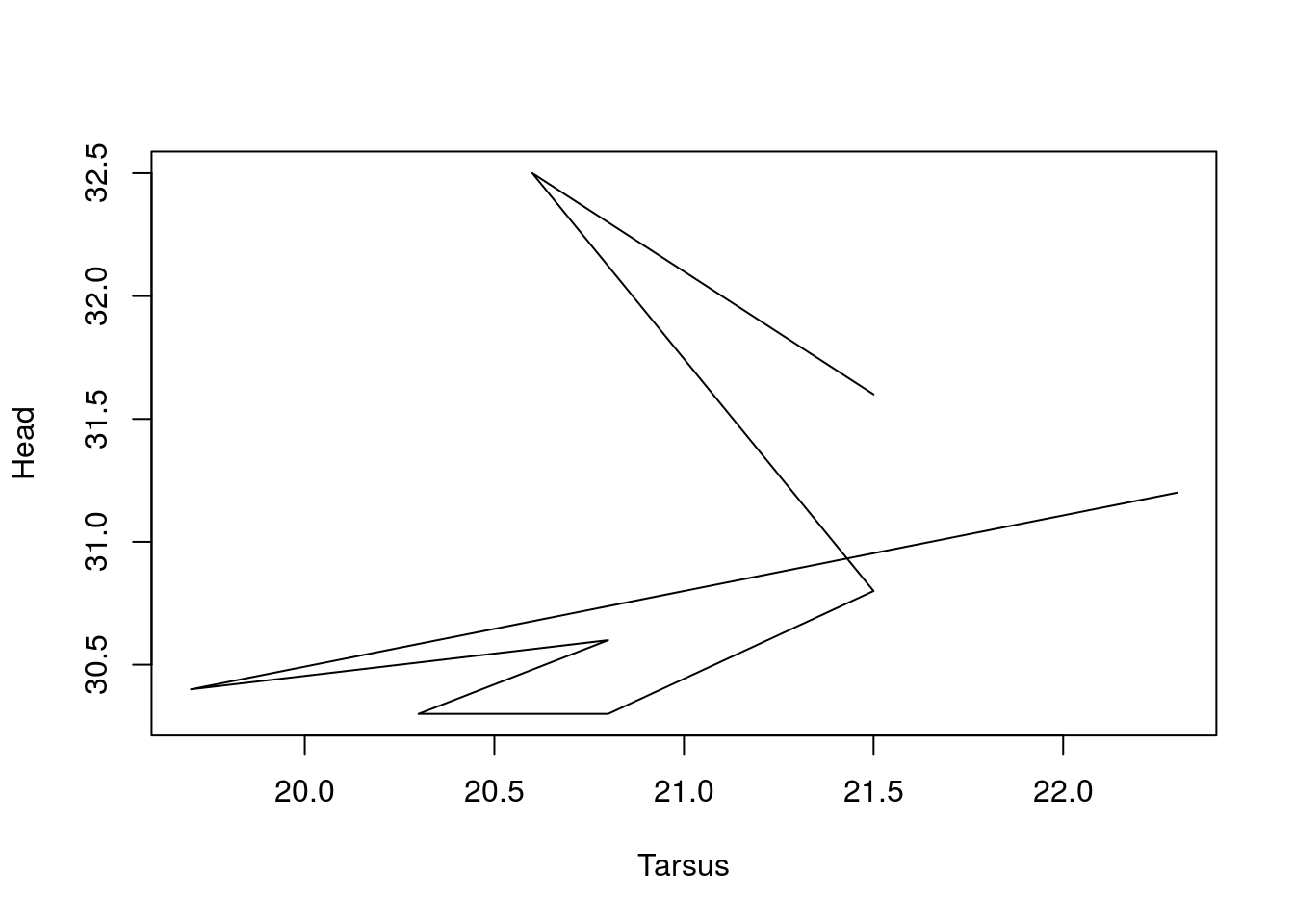The line goes from point to point, in the order that the x and y coordinates appear in the dataframe. Now try a graph of points joined by lines in between.

``plot(Head ~ Tarsus, data = BirdData, type = 'b')``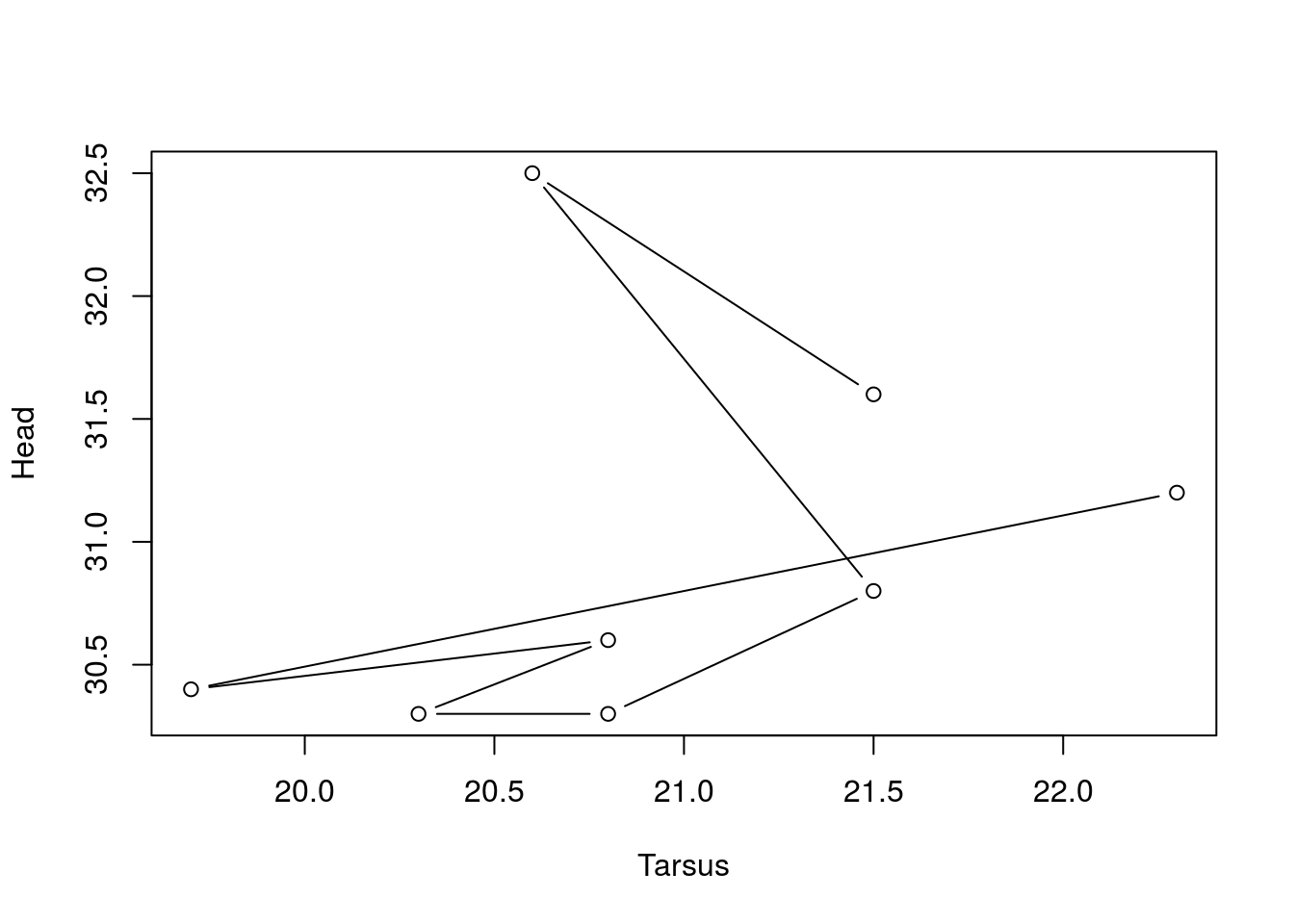We can add text to this figure to describe the x and y-axes, as well as give the plot a title. The arguments `xlab =`, `ylab =`, and `main =` correspond to these parts of the graph, and all take text strings in quotes.

For a graph of sparrow head size as a function of species, set xlab to ‘Species of Sparrow’, ylab to ‘Head Size (mm)’, and the graph title to ‘A Boxplot of Sparrows’.

``plot(Head ~ Species, data = BirdData, xlab = 'Species of Sparrow', ylab = 'Head Size (mm)', main = 'A Boxplot of Sparrows')``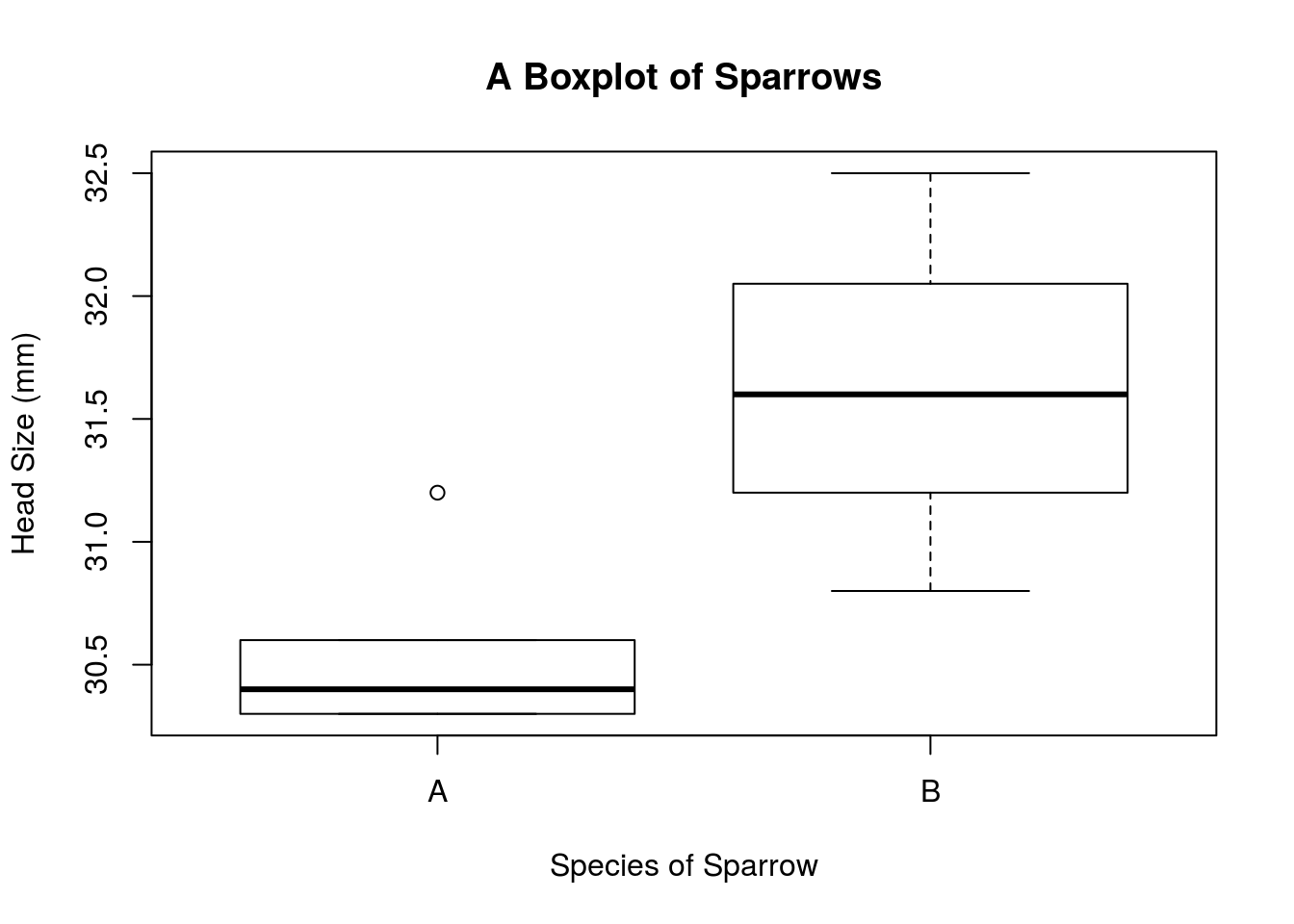A further thing you might wish to modify is the plotting characters themselves. Maybe empty circles is just not doing it for you.

This figure illustrates some of the plotting characters available to you. They are called by number, using the argument `pch =`. Characters 21:25 can have two different colours specified and can be filled with a colour different from the line. We will look at colour in the next lesson.

``````plot(   x = c(rep(1,5),rep(2,5),rep(3,5),rep(4,5),rep(5,5)),
y = rep(5:1,5),
axes = F,
xlab = "",ylab = "",
xlim = c(1,6),ylim = c(1,5),
pch = 1:25,
bg = "red",cex = 3)
text(   x = c(rep(1,5),rep(2,5),rep(3,5),rep(4,5),rep(5,5))+.5,
y = rep(5:1,5),
labels = as.character(1:25))
abline(h = (1:5)+0.5,col = "grey")
abline(v = (1:5)+0.75,col = "grey")``````